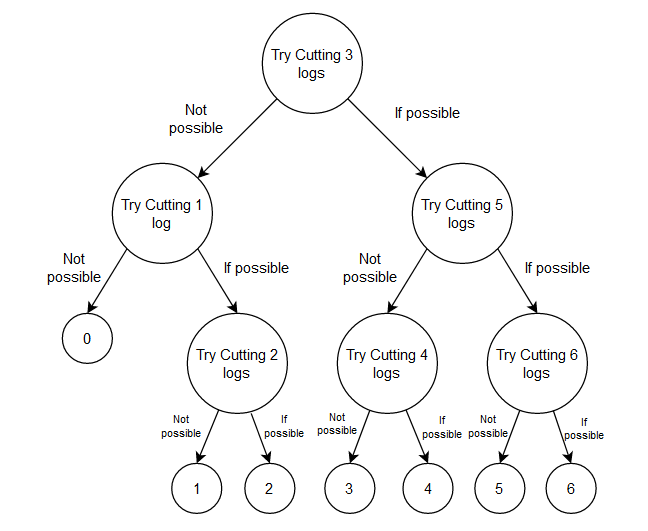# Cut Logs

Posted: 19 Dec, 2020
Difficulty: Hard

## PROBLEM STATEMENT

#### Can you calculate the minimum number of moves in which Ninja can know the limit of axes?

##### Some points to notice about axes are:
``````1. An axe that is used to cut a lesser or equal number of logs than its limit can be used again.

2. An axe that is used to cut more logs than its limit will be broken. Thus, it cannot be used again.

3. All the axes have the same limit of cutting logs until broken.

4. An axe may also cut N logs or may not even cut a single log.
``````

#### Example

``````Let the number of axes (K) be 2 & the capacity of the log cutting stand (N) be 6.
````````````From the above example, we can see that the maximum number of moves is 3 for 2 axes and a capacity of 6 logs.
``````
##### Input format:
``````The first line of input contains an integer ‘T’ denoting the number of queries or test cases.

The first and only line of each input consists of 2 space-separated integers ‘K’ and ‘N’ denoting the number of axes and the capacity of log cutting stand simultaneously.
``````
##### Output format:
``````For each test case, print the minimum number of moves required to know the limit of the axe.

Print the output of each test case in a separate line.
``````
##### Note:
``````You do not need to print anything, it has already been taken care of. Just implement the given function.
``````
``````Can you solve this in the worst-case time complexity of N ^ (1/3)
``````
##### Constraints:
``````1 <= T <= 10
1 <= K <= 100
1 <= N <= 10000
``````Approach 1

The very first approach can be to try cutting logs and find the one with the minimum number of moves.

1. The idea is to use recursion to reduce the big problem into several small subproblems.
2. We will try to cut each possible number of logs and get the answer for checking the remaining number of logs.
3. The algorithm for the following will be:

cutLogs( K, N ):
• If N <= 1 or K <= 1, return N.
• Initialize a variable ans = N
• Loop for ( i=1; i <= N; i++ ):
• Initialize cur = max of
• cutLogs(K-1, i-1), (If axe couldn’t cut i logs)
• cutLogs(K, N-i) ( If axe could cut i logs)
• Minimize the answer i.e. ans = min( ans, cur )